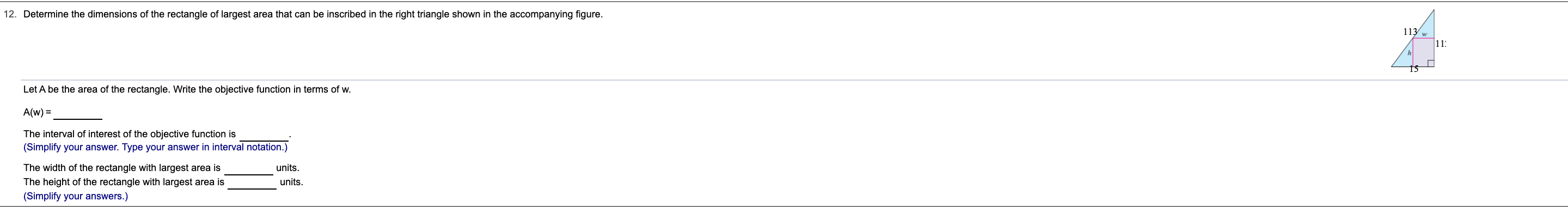# 12. Determine the dimensions of the rectangle of largest area that can be inscribed in the right triangle shown in the accompanying figure. 113 w 11 Let A be the area of the rectangle. Write the objective function in terms of w A(w) The interval of interest of the objective function is (Simplify your answer. Type your answer in interval notation.) The width of the rectangle with largest area is units. The height of the rectangle with largest area is units. (Simplify your answers.)

Question

Can I get help with this problem step by step?help_outlineImage Transcriptionclose12. Determine the dimensions of the rectangle of largest area that can be inscribed in the right triangle shown in the accompanying figure. 113 w 11 Let A be the area of the rectangle. Write the objective function in terms of w A(w) The interval of interest of the objective function is (Simplify your answer. Type your answer in interval notation.) The width of the rectangle with largest area is units. The height of the rectangle with largest area is units. (Simplify your answers.) fullscreen

### Want to see this answer and more?

Experts are waiting 24/7 to provide step-by-step solutions in as fast as 30 minutes!*

*Response times may vary by subject and question complexity. Median response time is 34 minutes for paid subscribers and may be longer for promotional offers.
Tagged in
Math
Calculus

### Derivative